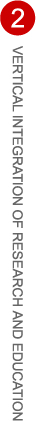# MATH 4800

## Fall 2007 course

Topic: Metric Spaces, The Contraction Mapping Principle, Fractals and Other Applications
Instructor: Klaus Schmitt
Catalog Number: MATH 4800-1
Credits: 3
Time/Location: MW, 2:00-3:20 PM, LCB 323

Registering requires obtaining the class number from the instructor. Prerequisites: Math 3210 & 3220, Foundations of Analysis I & II

The contraction mapping principle is one of the most useful tools in the study of nonlinear equations. It is a fixed point theorem which guarantees that a contraction mapping of a complete metric space to itself has a unique fixed point which may be obtained as the limit of an iteration scheme defined by repeated images under the mapping of an arbitrary starting point in the space. As such, it is a constructive fixed point theorem and, hence, may be implemented for the numerical computation of the fixed point.

The course will be devoted to a study of metric spaces, various abstract forms of the contraction mapping principle and applications of these ideas to the study of fractals, dynamical systems and differential equations.
Metric Spaces: Examples of metric spaces; Normed linear spaces; Hausdorff metric; Hausdorff and other dimensions; Hilbert's projective metric.

The Contraction Mapping Principle: The principle; Banach fixed point theorem; Newton's method; the Implicit and Inverse Function Theorems; the theorems of Perron-Frobenius and of Krein-Rutman.

Applications: Similarity transformations and iterated function systems; the space where fractals live; fractal dimensions; scaling laws; invariant measures; dynamical systems and PDE's.
Tuition Benefit:
The NSF VIGRE program provides a small tuition benefit for US students (US citizens and permanent residents). Details will be provided once total enrollment has been determined.

Past Courses:
Fall 2006, Math Finance
Spring 2007, Fractals